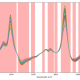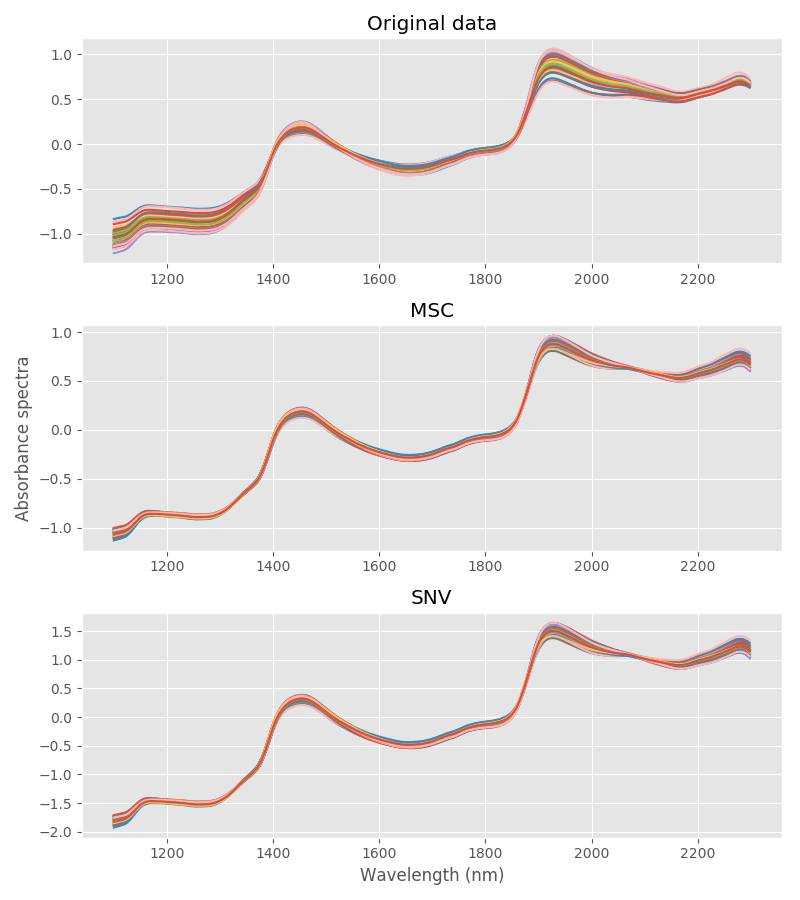###### A variable selection method for PLS in Python
07/04/2018###### Exporting NIR regression models built in Python
08/19/2018Ever wanted to master the art of NIR calibration but got lost in the acronyms? Struggling with getting simple explanations of the basics? Well then, this post is for you and today we’ll work through two scatter correction techniques for NIR spectroscopy in Python. We’ll discuss functions to perform Multiplicative Scatter Correction (MSC) and Standard Normal Variate (SNV) on near-infrared (NIR) data. Three acronyms in one sentence, that’s a good start.

Data pre-processing is an essential step to build most (ahem, all) types of calibration models in NIR analysis. With a well-designed pre-processing step, the performance of the model is greatly improved.

Before we move on, you want to take a look at some of our past post on the topic, here you go.

Pre-processing techniques can be generally divided into 1) Scatter Corrections techniques and 2) Derivative techniques. Both MSC and SNV belong to the first category.

In this post we are going to introduce the problem of scatter correction, describe the details and write some code to perform both MSC and SNV, then apply both method on some real data.

# Correcting scattering effects in NIR

NIR spectra contain a mix of diffuse and specular reflectance (or straight transmittance).  Three main factors affecting the shape of each spectrum are:

1. Different wavelengths of the incident light experience different absorption by the sample, due to the chemical nature of the sample itself. In most cases this is the signal we want to measure, and it relates to the analyte of interest.
2. Differences in particle size in the material will cause light to be deviated at different angles depending on it wavelength. Scattering effects (particle size), along with possible differences in path length constitute the major cause of variations in NIR spectra.
3. Path length differences from sample to sample due to positioning and/or irregularities in the sample surface.

Scattering effects can be both additive and multiplicative. Additive effects (such as path length differences) produce a baseline displacement of the spectrum along the vertical axis, while multiplicative effects (particle size for instance)  modify the local slope of the spectrum.

The idea behind scattering corrections is to get rid of all effects that are unrelated to the chemical nature of the sample, but just depends on the sample morphology and measurement geometry.  So, the idea goes, if we are able to get rid of these undesirable effects beforehand, we should get a better model for the quantity of interest.

As always, that is easier said than done. In practice it may be extremely difficult to separate scattering from absorbance effects, and the methods developed by the community tend to be an approximation that is valid under some assumptions.

Having said that however, years of practice showed that both MSC and SNV often do a good job in improving the quality of the calibration model and indeed are two of the most common, yet simple, pre-processing techniques for NIR data.

Let’s dive into it!

# Multiplicative Scatter Correction in Python

MSC requires a reference spectrum to work. This is the most important difference between MSC and SNV. The reference spectrum is ideally a spectrum free of scattering effects.

Now, as you can gather, getting our hands on a spectrum that is free of unwanted scattering effects is not easy, definitely not across all wavelengths we are interested in. For this reason, if the data is reasonably well behaved, we can take the average spectrum to be a close approximation to an ideal spectrum. Particle size and path length effects should vary randomly from sample to sample, and therefore the average should reasonably reduce these effects, at least that’s what we hope. This is the main assumption behind MSC.

Mathematically, if we call $$X_{m}$$ the mean spectrum, the correction is done in two steps.

1. First we regress each spectrum $$X_{i}$$  against the mean spectrum. This is done by ordinary least squares. $$X_{i} \approx a_{i} + b_{i}X_{m}$$ .
2. We calculate the corrected spectrum $$X^{\mathrm{msc}}_{i} = (X_{i}-a_{i})/b_{i}$$.

In general all these spectra have a non-zero mean, and therefore we can optionally mean centre the spectra beforehand.

Here’s a Python function to perform MSC.

Note that, in addition to the corrected spectra, it is good practice to return the mean spectrum too. That can be used on a second data set (for instance validation data) as a reference correction that is consistent with the first set of spectra.

# Standard Normal Variate in Python

Unlike MSC, SNV correction is done on each individual spectrum, and a reference spectrum is not required. The SNV correction can be divided in two conceptual steps as well.

1. Mean centre each spectrum $${X}_{i}$$ by taking away its mean $$\bar{X}_{i}$$
2. Divide each mean centred spectrum by its own standard deviation:  $$X^{\mathrm{snv}}_{i} = (X_{i}-\bar{X}_{i})/\sigma_{i}$$.

Translated into Python the previous algorithm looks something like this

# Putting it all together

To test these functions, I’ve run the following code

The data set is the same that I’ve used in past articles. It comes from a NIR reflectance experiment on peaches taken with the Brimrose Luminar 5030. The output isIndeed the two methods give an almost identical result, at least in this case. As it has been first shown by Danoha et al. in this paper (if you can’t get a copy, feel free to contact me), the two methods are indeed related by a linear transformation.

In closing, one of the advantage of MSC is that can relate all spectra to a common reference. If the reference spectrum is, to a good approximation, close to a spectrum free of unwanted scattering, this is a pretty good choice. Conversely, if outliers are present then the mean spectrum may not be a good reference and the MSC is not a good choice. In these cases SNV can be opted for instead.

Thanks for reading and until next time!##### Daniel Pelliccia
Physicist and an entrepreneur. Founder and Managing Director at Instruments & Data Tools, specialising in optical design and analytical instrumentation. Founder at Rubens Technologies, the intelligence system for the fresh fruit export industry.